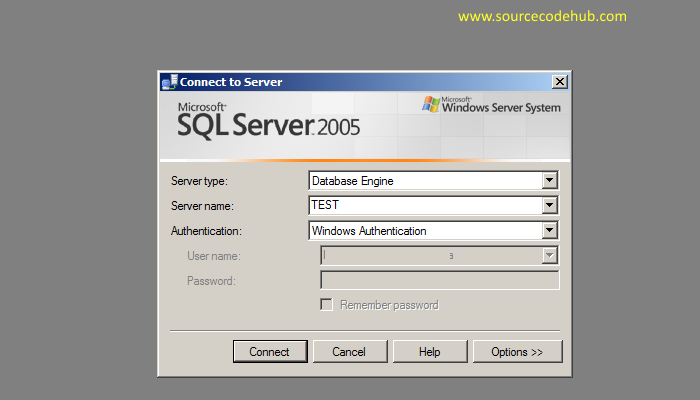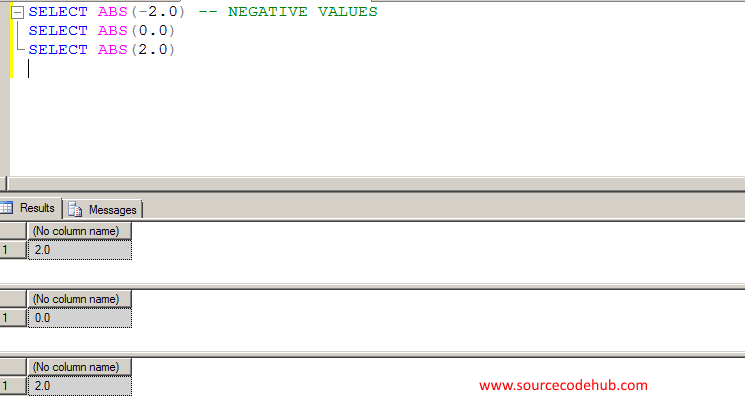# how can we convert negative a number to positive number in SQL Server 2005/2008.

Category > MSSQLSERVER || Published on : Sunday, July 27, 2014 || Views: 16487 || ABS function negative a number to a positive number use ABS function

If we wan to convert a number to positive number then we can use the ABS function hwich will convert the negative number to positive one.

Follow the following below steps to convert a negative a number to positive number in SQL Server 2005/2008

Step1: Open the SQL Server 2008 EditionStep 2: Create a new script(test databse or select any pre created database for the testing purpose )Step 3: Write the following SQL statement, I have used the ABS function there to a number to positive number in SQL Server 2005/2008

```SELECT ABS(-2.0) -- NEGATIVE VALUES
SELECT ABS(0.0)
SELECT ABS(2.0)
```

Step 4: Output as we needed.so guys, we have learned in this tutorial, how we can convert negative a number to positive number in SQL Server 2005/2008.